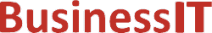O nás     Inzerce     KontaktSpolehlivé informace o IT již od roku 2011[Linux manuál]

# asin, asinf, asinl: funkce oblouku

Originální popis anglicky: asin, asinf, asinl - arc sine function

Návod, kniha: POSIX Programmer's Manual

## STRUČNĚ

#include <math.h>

double asin(double x);

float asinf(float x);

long double asinl(long double x);

## POPIS / INSTRUKCE

These functions shall compute the principal value of the arc sine of their argument x. The value of x should be in the range [-1,1].
An application wishing to check for error situations should set errno to zero and call feclearexcept(FE_ALL_EXCEPT) before calling these functions. On return, if errno is non-zero or fetestexcept(FE_INVALID | FE_DIVBYZERO | FE_OVERFLOW | FE_UNDERFLOW) is non-zero, an error has occurred.

## NÁVRATOVÁ HODNOTA

Upon successful completion, these functions shall return the arc sine of x, in the range [-pi/2,pi/2] radians.
For finite values of x not in the range [-1,1], a domain error shall occur, and  either a NaN (if supported), or  an implementation-defined value shall be returned.
If x is NaN, a NaN shall be returned.
If x is ±0, x shall be returned.
If x is ±Inf, a domain error shall occur, and either a NaN (if supported), or an implementation-defined value shall be returned.
If x is subnormal, a range error may occur and x should be returned.

## CHYBY / ERRORY

These functions shall fail if:
Domain Error
The x argument is finite and is not in the range [-1,1],  or is ±Inf.
If the integer expression (math_errhandling & MATH_ERRNO) is non-zero, then errno shall be set to [EDOM]. If the integer expression (math_errhandling & MATH_ERREXCEPT) is non-zero, then the invalid floating-point exception shall be raised.

These functions may fail if:
Range Error
The value of x is subnormal.
If the integer expression (math_errhandling & MATH_ERRNO) is non-zero, then errno shall be set to [ERANGE]. If the integer expression (math_errhandling & MATH_ERREXCEPT) is non-zero, then the underflow floating-point exception shall be raised.

The following sections are informative.

None.

## APPLICATION USAGE

On error, the expressions (math_errhandling & MATH_ERRNO) and (math_errhandling & MATH_ERREXCEPT) are independent of each other, but at least one of them must be non-zero.

None.

None.

## SOUVISEJÍCÍ

feclearexcept() , fetestexcept() , isnan() , sin() , the Base Definitions volume of IEEE Std 1003.1-2001, Section 4.18, Treatment of Error Conditions for Mathematical Functions, <math.h> Portions of this text are reprinted and reproduced in electronic form from IEEE Std 1003.1, 2003 Edition, Standard for Information Technology -- Portable Operating System Interface (POSIX), The Open Group Base Specifications Issue 6, Copyright (C) 2001-2003 by the Institute of Electrical and Electronics Engineers, Inc and The Open Group. In the event of any discrepancy between this version and the original IEEE and The Open Group Standard, the original IEEE and The Open Group Standard is the referee document. The original Standard can be obtained online at http://www.opengroup.org/unix/online.html .
 2003 IEEE/The Open Group
©2011-2020 BusinessIT.cz, ISSN 1805-0522 | Názvy použité v textech mohou být ochrannými známkami příslušných vlastníků.
Provozovatel: Bispiral, s.r.o., kontakt: BusinessIT(at)Bispiral.com | Inzerce: Best Online Media, s.r.o., zuzana@online-media.cz
O vydavateli | Pravidla webu BusinessIT.cz a ochrana soukromí | pg(9096)